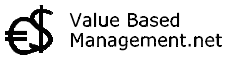## Return On Equity# ROE

Accounting valuation: Summary of Return On Equity (ROE). Abstract

Accounting Value

Single period measurementReturn On Equity (ROE) is an accounting valuation method similar to Return on Investment (ROI).

Because the numerator (Net Income) is an unreliable corporate performance measurement, the outcome of the formula for ROE must also be unreliable to determine success or corporate value.

However the formula keeps showing up in many annual reports still.

The degree to which Return On Equity (ROE) overstates the economic value depends on at least 5 factors:

1. length of project life (the longer, the bigger the overstatement)

2. capitalization policy (the smaller the fraction of total investment capitalized in the books, the greater will be the overstatement)

3. The rate at which depreciation is taken on the books (depreciation rates faster than straight-line basis will result in a higher ROE)

4. The lag between investment outlays and the recoupment of these outlays from cash inflows (the greater the time lag, the greater the degree of overstatement)

5. the growth rate of new investment (faster growing companies will have lower Return On Equity)

On top of this, ROE is sensitive to leverage: assuming that proceeds from debt financing can be invested at a return greater than the borrowing rate, ROE will increase with greater amounts of leverage.

### - formula Return on Equity calculation -

Net Income / Book Value of Shareholders' Equity = ROE

Book: Steven M. Bragg - Business Ratios and Formulas : A Comprehensive Guide

Book: Ciaran Walsh - Key Management Ratios

T I P : Here you can discuss and learn a lot more about asset valuation and Return On Equity.

Compare with Return on EquityEBIT  |  EBITDA  |  Economic Value Added  |  Cash Ratio  |  Current Ratio  |

 ©2022 Value Based Management.net - All names ™ by their owners